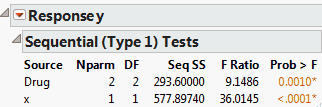Publication date: 05/24/2021

## Sequential Tests

The Sequential Tests report shows sums of squares and tests as effects are added to the model sequentially. The order of entry is defined by the order of effects as they appear in the Fit Model launch window’s Construct Model Effects list. The report in Figure 3.25 is for the Drug.jmp sample data table.

Figure 3.25 Sequential Tests ReportThe sums of squares that form the basis for sequential tests are also called Type I Sums of Squares. They are computed by fitting models in steps following the specified entry order of effects. Consider a specific effect. Compute the model sum of squares for a model containing all effects entered prior to that effect. Then compute the model sum of squares for a model containing those effects and the specified effect. The sequential sum of squares for the specified effect is the increase in the model sum of squares.

Refer to Figure 3.25, showing sequential sums of squares for the Drug.jmp sample data table. In the Fit Model launch window, Drug was entered first, followed by x. A model consisting only of Drug has model sum of squares equal to 293.6. When x is added to the model, the model sum of squares becomes 871.4974. The increase of 577.8974 is the sequential sum of squares for x.

The tests shown in the Sequential (Type 1) Tests report are F tests based on sequential sums of squares, also called Type I Tests. The F Ratio tests the specified effect, where the model contains only that effect and the effects listed above it in the Source column.

The sequential sums of squares sum to the model sum of squares. Another nice feature is that, under the usual model assumptions, the values are statistically independent of each other. However, they do depend on the order of terms in the model and, as such, are not appropriate in many situations.

Sequential tests are considered appropriate in the following situations:

balanced analysis of variance models specified in proper sequence (that is, two-way interactions follow main effects in the effects list, and so on)

purely nested models specified in the proper sequence

polynomial regression models specified in the proper sequence.

The tests given in the Parameter Estimates and Effect Tests reports are based on Type III Sums of Squares. Here the sum of squares for an effect is the extra sum of squares explained by the effect after all other effects have been entered in the model.